Question

# Calculate the equilibrium constant from the standard free energy change. Using standard thermodynamic data (linked), calculate...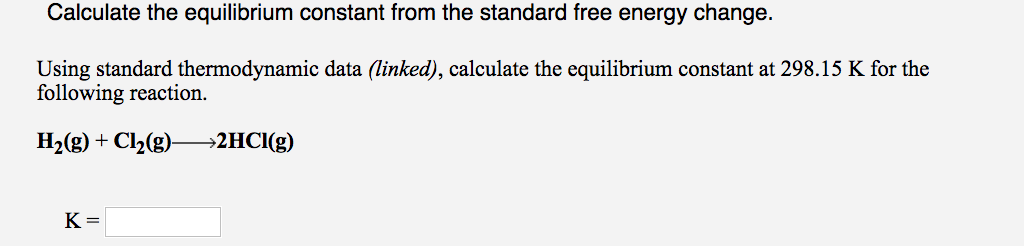Calculate the equilibrium constant from the standard free energy change. Using standard thermodynamic data (linked), calculate the equilibrium constant at 298.15 K for the following reaction. H2(g) + Cl2(g)—>2HCI(g) K=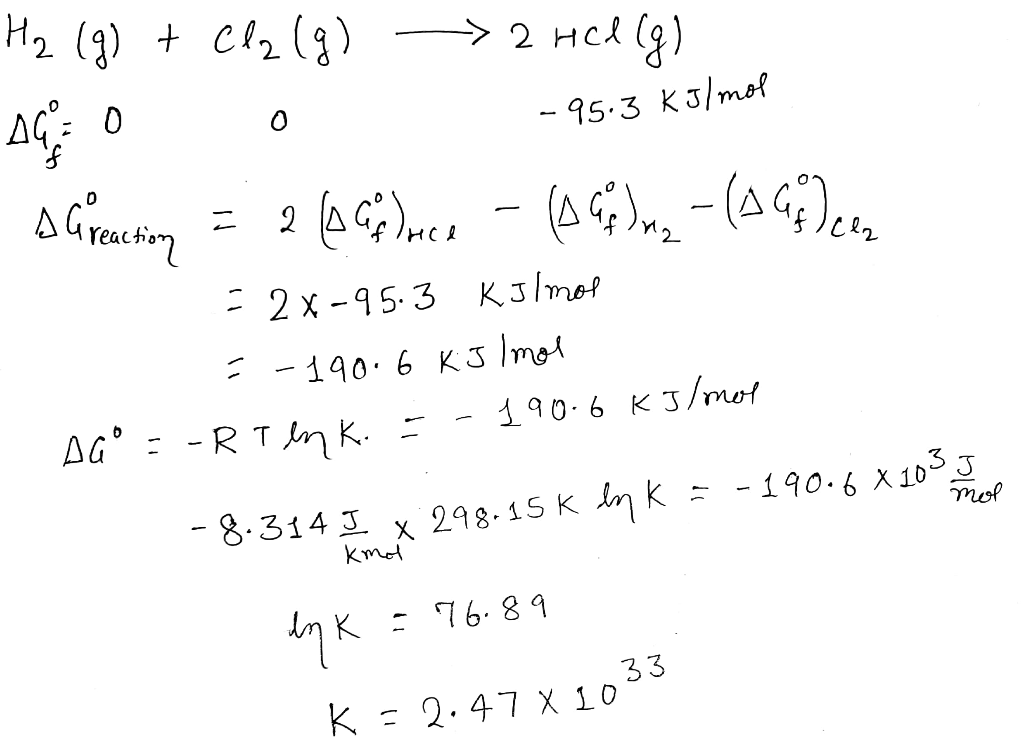#### Earn Coins

Coins can be redeemed for fabulous gifts.

Similar Homework Help Questions
• ### Calculate the equilibrium constant from the standard free energy change. Using standard thermodynamic data (linked), calculate...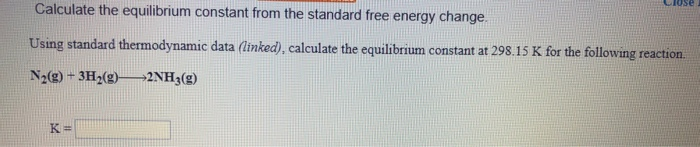Calculate the equilibrium constant from the standard free energy change. Using standard thermodynamic data (linked), calculate the equilibrium constant at 298.15 K for the following reaction. N2(g) + 3H2(9) 2NH3(g)

• ### Calculate the equilibrium constant from the standard free energy change. Using standard thermodynamic data (linked), calculate...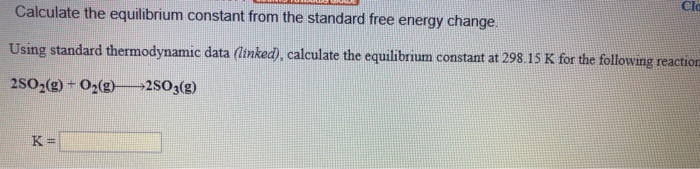Calculate the equilibrium constant from the standard free energy change. Using standard thermodynamic data (linked), calculate the equilibrium constant at 298.15 K for the following reaction 2502(g) + O2(0) 2503(9) AH°F (kJ/mol) AGⓇ, (kJ/mol sº (/mol K) 205.1 Oxygen 02(0) (9) O3(9) 249.2 231.7 161.1 142.7 163.2 238.9 AH°: (kJ/mol Agºr (kJ/mol) 5° (J/mol K) 31.8 0.3 0.3 278.8 3 3.0 167.8 33.0 - 15.0 Sulfur S(s, rhombic) S(s, monodinic) S(g) Sz"(aq) Sg(9) SzCl2(g) SF6(9) H2S(9) soz(9) SO3(0) 1 0....

• ### Tutored Practice Problem 19.3.4 COUNTS TOWARDS GRADE Calculate the equilibrium constant from the standard free energy...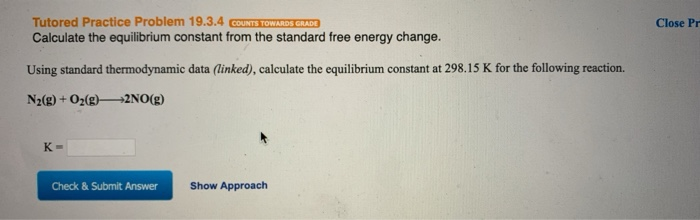Tutored Practice Problem 19.3.4 COUNTS TOWARDS GRADE Calculate the equilibrium constant from the standard free energy change. Close Pr Using standard thermodynamic data (linked), calculate the equilibrium constant at 298.15 K for the following reaction. N2(g) + O2(g) +2NO(g) K- Check & Submit Answer Show Approach

• ### Using standard thermodynamic data (linked), calculate the equilibrium constant at 298.15 K for the following reaction....

Using standard thermodynamic data (linked), calculate the equilibrium constant at 298.15 K for the following reaction. HCl(g) + NH3(g)--->NH4Cl(s)      K =

• ### Calculate the free energy change under nonstandard state conditions. Close Problem Use standard thermodynamic data (in...

Calculate the free energy change under nonstandard state conditions. Close Problem Use standard thermodynamic data (in the Chemistry References) to calculate G at 298.15 K for the following reaction, assuming that all gases have a pressure of 13.54 mm Hg. CO(g) + Cl2(g)COCl2(g) G = kJ/mol

• ### help with these please Fe2O3(5) + 3H2(g) +2Fe(s) + 3H2O(g) Using standard thermodynamic data at 298K,...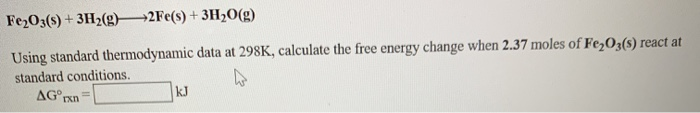help with these please Fe2O3(5) + 3H2(g) +2Fe(s) + 3H2O(g) Using standard thermodynamic data at 298K, calculate the free energy change when 2.37 moles of Fe2O3() react at standard conditions. ho AG rxnk 2CO(g) + O2(g) +2CO2(g) Using standard thermodynamic data at 298K, calculate the free energy change when 1.540 moles of CO(g) react at standard conditions AGºran 3 Fe2O3(-) + H2(g) 2 Fe3O4(5) + H2O(g) U ng the standard thermodynamic data in the tables linked above, calculate the equilibrium...

• ### 1.) Consider the reaction: Fe3O4(s) + 4H2(g)3Fe(s) + 4H2O(g) Using standard thermodynamic data at 298K, calculate...

1.) Consider the reaction: Fe3O4(s) + 4H2(g)3Fe(s) + 4H2O(g) Using standard thermodynamic data at 298K, calculate the free energy change when 2.48 moles of Fe3O4(s) react at standard conditions.      G°rxn = kJ 2.)Consider the reaction: H2(g) + Cl2(g)2HCl(g) Using standard thermodynamic data at 298K, calculate the free energy change when 1.750 moles of H2(g) react at standard conditions.    G°rxn = kJ

• ### Consider the reaction: H2(g) + F2(g)2HF(g) Using standard thermodynamic data at 298K, calculate the free energy...

Consider the reaction: H2(g) + F2(g)2HF(g) Using standard thermodynamic data at 298K, calculate the free energy change when 2.410 moles of H2(g) react at standard conditions. G°rxn = ___ kJ

• ### Calculate the change in Gibbs free energy at standard conditions (TSS) and the equilibrium constant for...

Calculate the change in Gibbs free energy at standard conditions (TSS) and the equilibrium constant for each of the following reactions. (a) CH4(g) + H2O(g) equilibrium reaction arrow CO(g) + 3 H2(g); ?H° = +205.9 kJ, ?S° = +214.7 J/K ?G° 141.9 Correct: Your answer is correct. kJ K (b) CaCO3(s) equilibrium reaction arrow CaO(s) + CO2(g); ?H° = +179.2 kJ, ?S° = +160.2 J/K ?G° 131.5 Correct: Your answer is correct. kJ K

• ### Using standard free energy of formation values given in the introduction, calculate the equilibrium constant Kp of the...

Using standard free energy of formation values given in the introduction, calculate the equilibrium constant Kp of the reaction Cl2(g)+2NO(g)?2NOCl(g) The standard free energy of the reaction represents the drive the reaction has under standard conditions to move toward equilibrium from point A to point X in the diagram. Express the equilibrium constant numerically using three significant figures.